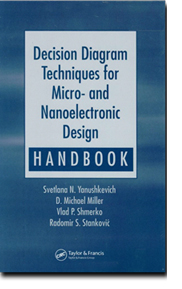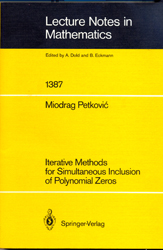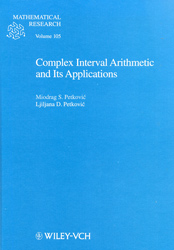### ОсталоYanushkevich, S.N.,   Miller, D.M., Shmerko, V.P., Stanković, R.S., Decision Diagram Techniques for Micro- and Nanoelectronic Design Handbook,  CRC Press, 2005,

ISBN-10: 0849334241

ISBN-13: 978-0849334245

Decision diagram (DD) techniques are very popular in the electronic design automation (EDA) of integrated circuits, and for good reason. They can accurately simulate logic design, can show where to make reductions in complexity, and can be easily modified to model different scenarios.

Presenting DD techniques from an applied perspective, Decision Diagram Techniques for Micro- and Nanoelectronic Design Handbook provides a comprehensive, up-to-date collection of DD techniques. Experts with more than forty years of combined experience in both industrial and academic settings demonstrate how to apply the techniques to full advantage with more than 400 examples and illustrations. Beginning with the fundamental theory, data structures, and logic underlying DD techniques, they explore a breadth of topics from arithmetic and word-level representations to spectral techniques and event-driven analysis. The book also includes abundant references to more detailed information and additional applications.

Decision Diagram Techniques for Micro- and Nanoelectronic Design Handbook collects the theory, methods, and practical knowledge necessary to design more advanced circuits and places it at your fingertips in a single, concise reference.

Stanković, R.S., Moraga, C., Astola, J.T., Fourier Analysis on Finite Groups with Applications in Signal Processing and System Design, Wiley-IEEE Press, 2005,
ISBN-10: 0471694630
ISBN-13: 978-0471694632

The majority of publications in spectral techniques consider Fourier transform on Abelian groups. However, non-Abelian groups provide notable advantages in efficient implementations of spectral methods.
Fourier Analysis on Finite Groups with Applications in Signal Processing and System Design examines aspects of Fourier analysis on finite non-Abelian groups and discusses different methods used to determine compact representations for discrete functions providing for their efficient realizations and related applications. Switching functions are included as an example of discrete functions in engineering practice. Additionally, consideration is given to the polynomial expressions and decision diagrams defined in terms of Fourier transform on finite non-Abelian groups.Stanković, R.S., Astola, J.T., Spectral Interpretation of Decision Diagrams, Springer, 2003

ISBN-13 978-0387955452

Decision diagrams (DDs) are data structures for efficient (time/space) representations of large discrete functions. In addition to their wide application in engineering practice, DDs are now a standard part of many CAD systems for logic design and a basis for severe signal processing algorithms. Spectral Interpretation of Decision Diagrams derives from attempts to classify and uniformly interpret DDs through spectral interpretation methods, relating them to different Fourier-series-like functional expressions for discrete functions and a group-theoretic approach to DD optimization. The book examines DDs found in literature and engineering practice and provides insights into relationships between DDs and different polynomial or spectral expressions for representation of discrete functions. In addition, it offers guidelines and criteria for selection of the most suitable representation in terms of space and time complexity. The work complements theory with numerous illustrative examples from practice. Moreover, the importance of DD representations to the verification and testing of arithmetic circuits is addressed, as well as problems related to various signal processing tasks.WELDING PROCESSES

Chapter 11

Analytical Model for Estimating the Amount of Heat Generated During Friction Stir Welding: Application on Plates Made of Aluminium Alloy 2024 T351

By Miroslav Mijajlović and Dragan Milčic

ISBN: 978-953-51-0854-2

Miroslav Mijajlović and Dragan Milčic (2012). Analytical Model for Estimating the Amount of Heat Generated During Friction Stir Welding: Application on Plates Made of Aluminium Alloy 2024 T351, Welding Processes, Dr. Radovan Kovacevic (Ed.), ISBN: 978-953-51-0854-2, InTech, DOI: 10.5772/53563.

Available from: http://www.intechopen.com/books/welding-processes/analytical-model-for-estimating-the-amount-of-heat-generated-during-friction-stir-welding-applicatioELSEVIER 2013

MULTIPOINT METHODS FOR SOLVING NONLINEAR EQUATIONS

Miodrag S. Petković, University of Niš, Serbia
Beny Neta, Naval Postgraduate School, Monterey, CA, USA
Ljiljana D. Petković, University of Niš, Serbia
Jovana Džunić, University of Niš, Serbia

ISBN: 978-0-12-397013-8

299 pages

Multipoint iterative methods belong to the class of the most efficient methods for solving nonlinear equations of the form f(x) = 0. Interest in multipoint methods has grown for two principal reasons. The first is that root solvers based on multipoint methods overcome theoretical limits of one point methods related to the convergence order and computational efficiency. Secondly, with the significant progress and developments made in computer hardware and software (multi-precision arithmetic and symbolic computation), implementation and convergence analysis of multipoint methods with the capability to generate root approximations of very high accuracy have become possible.
This book is the first on the topic and explains the most cutting-edge methods needed for precise calculations and explores the development of powerful algorithms to solve research problems. Multipoint methods have an extensive range of practical applications significant in research areas such as signal processing, analysis of convergence rate, fluid mechanics, solid state physics, and many others.
This book offers the reader both a systematic introduction to techniques for developing multipoint methods and a unified presentation of the multipoint iterative methods constructed during the last fifty years. The results presented in the book mainly reflect the research conducted over the past decade, and are devoted to multipoint methods that attain maximal order of convergence with a fixed number of function evaluations.
Intended as a combination of theoretical results, algorithmic aspects and symbolic computation, Multipoint Methods for Solving Nonlinear Equations serves as a text for students in math and applied math courses. It is also a reliable, well-structured professional reference for numerical analysts, engineers, physicists and computer scientists.SPRINGER-VERLAG, Lecture Notes in Mathematics 1387, 1989

ITERATIVE METHODS FOR SIMULTANEOUS INCLUSION OF POLYNOMIAL ZEROS

Miodrag S. Petković, University of Niš, Serbia

ISBN: 3-540-51486-6

263 pages

The simultaneous inclusion of polynomial complex zeros is a crucial problem in numerical analysis. Rapidly converging algorithms are presented in these monograph, including convergence analysis in terms of circular regions, and in complex arithmetic. Parallel circular iterations, where the approximations to the zeros have the form of circular regions containing these zeros, are efficient because they also provide error estimates. There are at present no book publications on this topic and one of the aims of this book is to collect most of the algorithms produced in the last 15 years.
To decrease the high computational cost of interval methods, several effective iterative processes for the simultaneous inclusion of polynomial zeros which combine the efficiency of ordinary floating-point arithmetic with the accuracy control that may be obtained by the interval methods, are set down, and their computational efficiency is described. The rate of these methods is of interest in designing a package for the simultaneous approximation of polynomial zeros, where automatic procedure selection is desired.
The book is both a text and a reference source for mathematicians, engineers, physicists and a computer scientists who are interested in new developments and applications, but the material is also accessible to anyone with graduate level mathematical background and some knowledge of basic computational complex analysis and programming.SPRINGER, Lecture Notes in Mathematics 1933, 2008

POINT ESTIMATION OF ROOT FINDING METHODS
Miodrag S. Petković, University of Niš, Serbia

ISBN: 978-3-540-77850-9

210 pages

This book sets out to state computationally verifiable initial conditions for predicting the immediate appearance of the guaranteed and fast convergence of iterative root finding methods. Attention is paid to iterative methods for simultaneous determination of polynomial zeros in the spirit of Smale's point estimation theory, introduced in 1986. Some basic concept and Smale's theory for Newton's method, together with its modifications and higher-order methods, are presented in the first two chapters. The remaining chapters contain the recent author's results on initial conditions guaranteing convergence of a wide class of iterative methods for solving algebraic equations. These conditions are of practical interest since they depend only on available data, the information of a function whose zeros are sought and initial approximations. The convergence approach presented can be applied in designing a package for the simultaneous approximations of polynomial zeros.WILEY-VCH, Mathematical Research, Vol. 105, 1998

COMPLEX INTERVAL ARITHMETIC AND ITS APPLICATIONS

Miodrag S. Petković, University of Niš, Serbia
Ljiljana D. Petković, University of Niš, Serbia

ISBN: 3-527-40134-2

284 pages

The aim of this monograph is to present formulas and methods developed using complex interval arithmetic. While most of numerical methods described in the literature deal with real intervals and real vectors, there is no systematic study of methods in complex interval arithmetic. The book fills this gap. Several main subjects are considered: outer estimates for the range of complex functions, especially complex centered forms, the best approximations of elementary complex functions by disks, iterative methods for the inclusion of polynomial zeros including their implementation on parallel computers, the analysis of numerical stability of iterative methods by using complex interval arithmetic and numerical computation of curvilinear integrals with error bounds. Mainly new methods are presented developed over the last years, including a lot of very recent results by the authors some of which have not been published before.
The book is both a text and a reference source for mathematicians, engineers, physicists, and computer scientists who are interested in new developments and applications, but material is also accessible to anyone with graduate level mathematical background and some knowledge of basic computational complex analysis and programming.